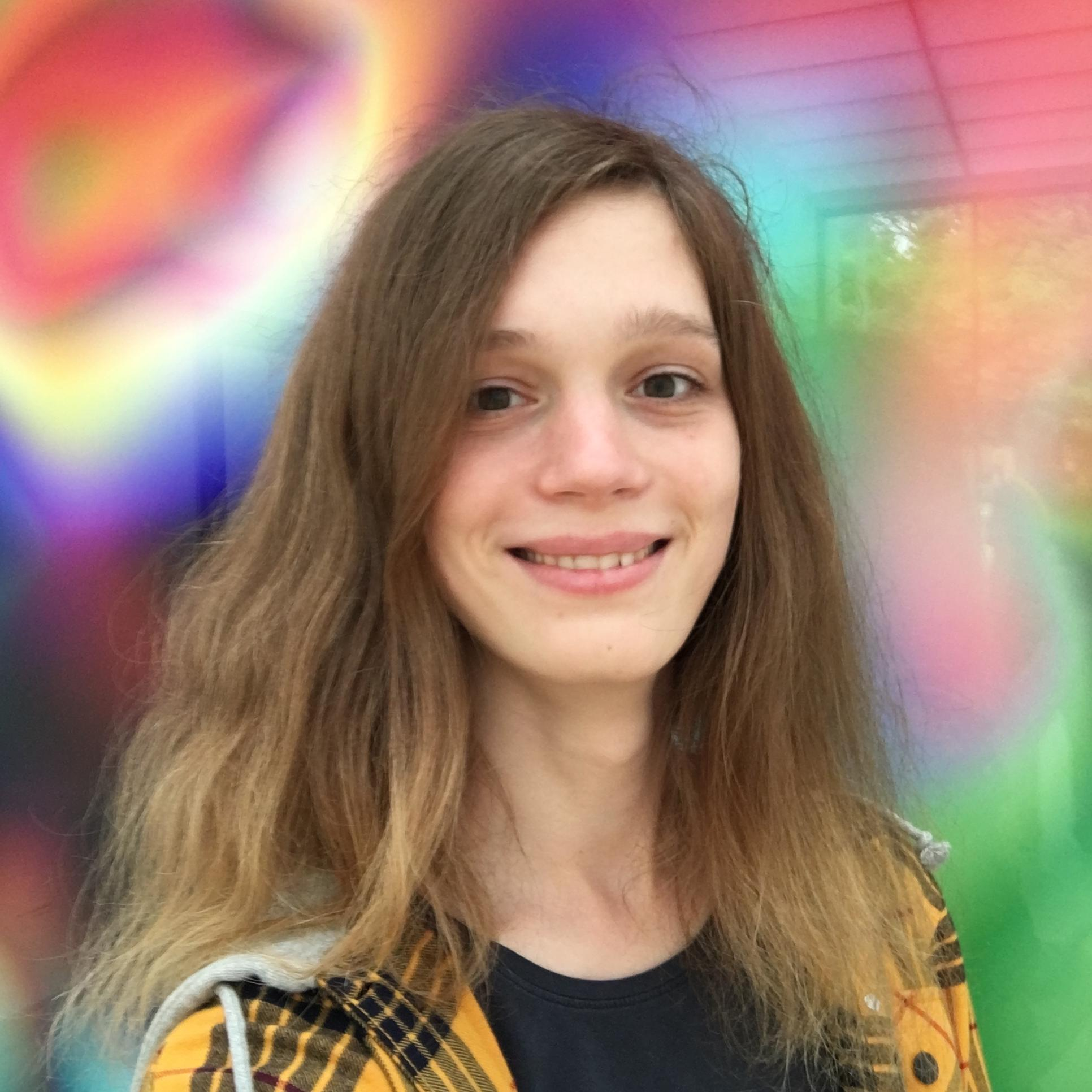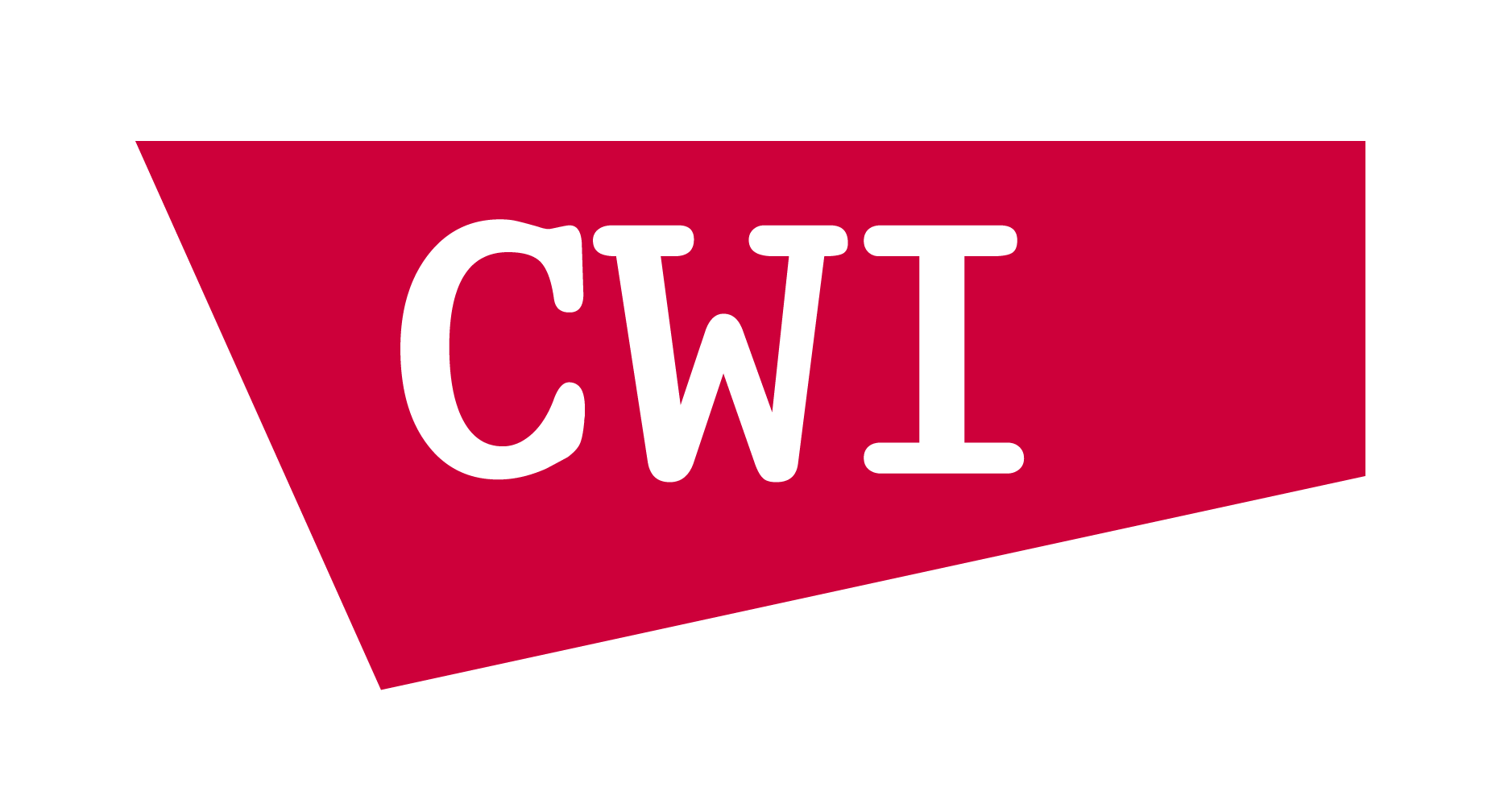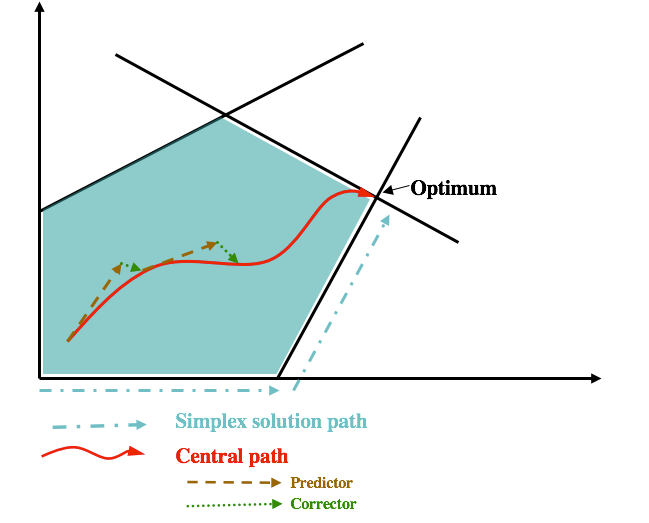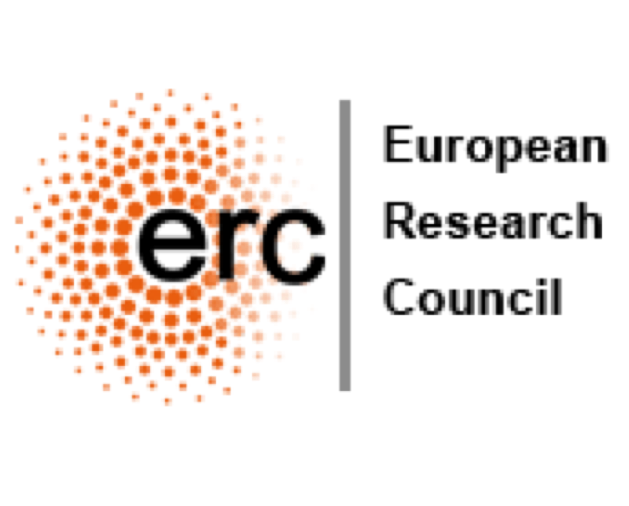Layered-Least-Squares
Interior Point Methods
a combinatorial optimization perspective
László Végh
London School of Economicsjoint work with
and
Bento Natura (LSE)
Aussois, 9 Jan 2020## Linear Programming

In standard form for $$A \in \mathbb{Q}^{m \times n}$$, $$b\in \mathbb{Q}^m$$, $$c\in \mathbb{Q}^n$$, \begin{aligned} \min \; &c^\top x & \max \; & y^\top b \\ Ax& =b & \qquad A^\top y + s &= c \\ x &\geq 0 & s & \geq 0 \\ \end{aligned}$## Timeline • ### Strongly polynomial algorithm for LP Smale's 9th question• ### Interior point methods Karmarkar • ### Ellipsoid Method Khachiyan • ### Simplex Method Dantzig • ### Origins Fourier ## Weakly vs Strongly Polynomial Algorithms for LP LP with $$n$$ variables, $$m$$ constraints $$L$$: encoding length of the input. weakly polynomial • $$\mathrm{poly}(m,n,L)$$ basic arithmetic operations. strongly polynomial • $$\mathrm{poly}(m,n)$$ basic arithmetic operations. • PSPACE: all numbers occurring in the algorithm must remain polynomially bounded in input size. Ellipsoid, interior point methods: running time bound heavily relies on $$L$$. ## Strongly Polynomial Algorithms for LP Network flow problems • Maximum flow: Edmonds–Karp–Dinitz '70-72 • Min-cost flow: Tardos '85 Special classes of LP • Feasibility of 2-variable-per-inequality systems: Megiddo '83 • Discounted Markov Decision Processes: Ye '05, Ye '11 • Maximum generalized flow problem: V. '17, Olver–V. '18 • ... ## Dependence on the constraint matrix only $$\min c^\top x,\, Ax=b,\, x\ge 0$$$ Running time dependent only on constraint matrix $$A$$, but not on $$b$$ and $$c$$.

General LP
• 'Combinatorial LPs'
If $$A$$ integral and $$|\mathrm{det}(B)| \leq \Delta$$ for all square submatrices of $$A$$, then LP solvable in $$\mathrm{poly}(m,n,\Delta)$$ arithmetic operations: Tardos '86
• 'Layered-least-squares (LLS) Interior Point Method'
LP solvable in $$O(n^{3.5}\log\bar\chi_A)$$ linear system solves: Vavasis–Ye '96

Condition number $$\bar\chi_A$$
• $$\bar\chi_A = O(2^{L_A})$$.
• Governs the stability of layered-least-squares solutions.
• Depends only on the subspace $$\ker(A)$$.
• NP-hard to approximate within a factor $$2^{\mathrm{poly}(\mathrm{rank}(A))}$$: Tunçel '99

## Scale invariance

##### \begin{aligned} \min \; &c^\top x & \max \; & y^\top b \\ Ax& =b & \qquad A^\top y + s &= c \\ x &\geq 0 & s & \geq 0 \\ \end{aligned}$$$A\in \R^{m\times n}$$. Let $$\mathbf{D}$$ denote the set of $$n\times n$$ positive diagonal matrices. • Since $$\bar\chi_A$$ only depends on $$\ker(A)$$, we have $$\bar\chi_A=\bar\chi_{RA}$$ for any nonsingular $$R\in\R^{m\times m}$$. • However, we may have $$\bar\chi_A\neq \bar\chi_{AD}$$ for $$D\in\mathbf{D}$$. • Diagonal rescaling (scaling) of the problem: Replace $$A'=AD$$, $$c'=Dc$$, $$b'=b$$ in the LP for $$D\in \mathbf{D}$$. • Corresponding variables transformation: $$x'=D^{-1} x$$, $$y'=y$$, $$s'=D s$$. • Central path is invariant under rescaling. • Standard interior point methods are also invariant under rescaling. • The Vavasis–Ye algorithm is not scaling invariant • $$O(n^{3.5} \log\bar\chi_A)$$ linear system solves [VY96]. ## Is there a scaling invariant LLS interior point method? $$\bar\chi_A^* := \inf\{\bar\chi_{AD}: D\in \mathbf{D}\}.$$$
• Vavasis–Ye (VY): $$O(n^{3.5} \log\bar\chi_A)$$ linear system solves.
• A scaling invariant version of VY would automatically give $$O(n^{3.5} \log\bar\chi_A^*)$$.
• The (scaling invariant) Mizuno–Todd–Ye Predictor-Corrector algorithm finds an $$\epsilon$$-approximate solution in time $$O(n^{3.5}\log\bar\chi_A^* + n^2\log\log(1/\varepsilon))$$: Monteiro–Tsuchiya '05.
• $$O(n^{3.5}\log\bar\chi_A^*)$$ iterations scaling invariant algorithm, but each iteration depends on the bit-complexity $$b$$ and $$c$$: Lan–Monteiro–Tsuchiya '09.

## Our contributions

A scaling invariant algorithm We give a scaling invariant LLS interior point method, settling the open question posed by Monteiro and Tsuchiya in '03.

Improved Runtime We show a running time bound of $$O(n^{2.5}\log(\bar\chi_A^\ast +n)\log n)$$ linear system solves. This is achieved via an improved analysis that also applies to the original VY algorithm.

Finding a nearly-optimal rescaling of $$A$$
• Tunçel '99: There exists no additive $${\mathrm{poly}(\mathrm{rank}(n))}$$-approximation of $$\log \bar\chi_A$$.

• The central path is the algebraic curve formed by $$\{w(\mu): \mu>0\}$$$• For $$\mu\to 0$$, the central path converges to an optimal solution $$w^*=(x^*,y^*,\mu^*)$$ ## The Mizuno-Todd-Ye Predictor Corrector algorithm• Start from point $$w^0=(x^0,y^0,s^0)$$ 'near' the central path at some $$\mu^0>0$$. • Alternate between two types of Newton steps. • Predictor steps: 'shoot down' the central path, decreasing $$\mu$$ by a factor at least $$1- \beta/\sqrt{n}$$. Move slightly 'farther' from the central path. • Corrector steps: do not change parameter $$\mu$$, but move back 'closer' to the central path. • Within $$O(\sqrt n)$$ iteration, $$\mu$$ decreases at least by a factor 2. ## Predictor Netwon step We obtain the step direction $$\Delta w=(\Delta x,\Delta y,\Delta s)$$ as solutions to $$\min\, \sum_{i=1}^n\left(\frac{x_i+\Delta x_i}{x_i}\right)^2 \quad \text{s.t. } A\Delta x = 0$$$

and
$$\min\, \sum_{i=1}^n\left(\frac{s_i+\Delta s_i}{s_i}\right)^2 \quad \text{s.t. } A^\top \Delta y + \Delta s = 0$$$Next iterate is obtained as $$w'=w+\alpha \Delta w = (x+\alpha\Delta x,y+\alpha \Delta y, s+\alpha\Delta s)$$$ for some $$\alpha\in [0,1]$$.
$$\alpha=1$$ would terminate with an optimal solution.

# Changes of $$x_i$$ and $$s_i$$ variables in the MTY Predictor-Corrector algorithm

## Recent weakly polynomial IPM successess

### A convenient characterization

For $$W=\ker(A)$$. Recall that $$\bar\chi_A$$ only depends on the subspace $$W$$.
Define the lifting map $$L_I^W : \mathrm{proj}_I(W) \to W$$ by $$L_I^W(v) := \argmin\left\{\|w\| : w_I = v, w \in W\right\}.$$$$$\quad\bar{\chi}_A =\max\left\{ \|L_I^W\|\, : {I\subseteq [n]}, I\neq\emptyset\right\}\, .$$$

## Properties of $$\bar\chi_A$$

$$\bar\chi_A=\sup\left\{\|A^\top \left(A D A^\top\right)^{-1}AD\|\, : D\in {\mathbf D}\right\}$$$We also use $$\bar \chi_W=\bar\chi_A$$ for the subspace $$W=\ker(A)$$. The following hold: 1. If the entries of $$A$$ are all integers, then $$\bar\chi_A$$ is bounded by $$2^{O(L_A)}$$, where $$L_A$$ is the input bit length of $$A$$. 2. $$\bar\chi_A = \max\left\{ \|B^{-1} A\| : B \text{ non-singular } m \times m \text{ submatrix of } A\right\}.$$$
3. $$\bar\chi_W=\bar\chi_{W^\perp}.$$## Application: Final rounding step in standard IPMs ##### \begin{aligned} \min \; &c^\top x & \max \; & y^\top b \\ Ax& =b & \qquad A^\top y + s &= c \\ x &\geq 0 & s & \geq 0 \\ \end{aligned}
• There exists a partition $$[n]=B^*\cup N^*$$ and optimal $$(x^*,y^*,s^*)$$ such that $$B^*=\mathrm{supp}(x^*),\, N^*=\mathrm{supp}(s^*)$$.
• Given $$w=(x,y,s)$$ close to the central path with 'small enough'
$$\mu=x^\top s/n$$, such that for each $$i\in [n]$$, either $$x_i\ll s_i$$ or $$x_i\gg s_i$$.
• We 'guess' $$B:=\{i: x_i\gg s_i\},\quad N:=\{i: x_i\ll s_i\}.$$$• We try to move to $$w'=w+\Delta w$$ such that $$\mathrm{supp}(x')\subseteq B$$, $$\mathrm{supp}(s')\subseteq N$$, $$x',s'\ge 0$$. • We need $$A\Delta x=0,\, \Delta x_N=-x_N,\, A^\top \Delta y+\Delta s=0,\, \Delta s_B=-s_B,$$$ and for $$x+\Delta x\ge0,\, s+\Delta s\ge 0$$ it suffices that $$\|\Delta x_B\|\leq \min_{i \in B} x_i,\, \|\Delta s_N\|\leq \min_{j \in N} s_j.$$• Natural choices are \begin{aligned} \Delta x&:=\arg\min\left\{ \|u\| : u_N=-x_N, A u=0\right\}\\ \Delta s&:=\arg\min\left\{\|v\| : v_B=-s_B, A^\top z +v=0\right\} \end{aligned}

## Approximating $$\kappa^*_A$$

[DHNV19+] $$\kappa^*_A=\max\left\{\prod_{(i,j)\in H} \kappa_{ij}^{1/|H|}:\, H \textrm{ is a cycle in the complete graph on }[n]\right\}$$$• Algorithmically, the optimal rescaling can be obtained via a minimum-mean cycle computation for the costs $$c_{ij}=-\log\kappa_{ij}$$. • Minor caveat: computing $$\kappa_{ij}$$ values is NP-complete • Luckily, for any $$g\in \ker(A)$$ with $$i,j\in \mathrm{supp}(g)\in \mathcal{C}$$, $$|g_j/g_i|\ge \kappa_{ij}/(\kappa^*_A)^2.$$$

## The Vavasis–Ye algorithm

### Learning the optimal partition of variables

• Assume the Predictor-Corrector method has already 'found' the partition $$[n]=B^*\cup N^*$$: $$x_i\gg x_j$$ and $$s_i\ll s_j$$ if $$i\in B^*$$, $$j\in N^*$$.
• A simple projection would find the optimal solution, but the usual predictor step does not: $$\min\, \sum_{i=1}^n\left(\frac{x_i+\Delta x_i}{x_i}\right)^2 \quad \text{s.t. } A\Delta x = 0$$$• What does the Vavasis–Ye algorithm do here? • First, solve $$\min, \sum_{\textcolor{red}{i\in N^*}}\left(\frac{x_i+\Delta \bar x_i}{x_i}\right)^2 \quad \text{s.t. } A\Delta \bar x = 0$$$ The $$N^*$$ components of the optimal $$\Delta\bar x$$ give $$\Delta x_{N^*}$$; in this case, $$\Delta x_{N^*}=0$$.
• Next, solve $$\min\, \sum_{\textcolor{red}{i\in B^*}}\left(\frac{x_i+\Delta \bar x_i}{x_i}\right)^2 \quad \text{s.t. } A\Delta \bar x = 0,\, \Delta \bar x_{N^*}=\Delta x_{N^*}$$\$

## Layering and crossover events in the Vavasis–Ye algorithm

• Arrange the variables into layers $$(J_1,J_2,\ldots,J_k)$$ as follows:
• Order $$x_1\ge x_2\ge\ldots\ge x_n$$.
• Start a new layer after $$x_i$$ whenever $$x_{i}>c\bar \chi_A x_{i+1}$$.
• Variables on lower layers 'barely influence' those on higher layers.
• Not scaling invariant!

### Progress measure

The variables $$x_i$$ and $$x_j$$ cross over between $$\mu$$ and $$\mu'$$ for $$\mu>\mu'>0$$, if
1. $$x_j\ge x_i/(\bar \chi_A)^n$$ at the iterate at $$\mu$$.
2. $$x_j(\mu'') < x_i(\mu'')/(\bar \chi_A)^n$$ for the central path point for any $$0<\mu''\le \mu'$$
In the Vavasis–Ye algorithm, a crossover event happens within every $$O(n\log \bar\chi_A)$$ iterations.
We do not know which two variables cross over!

## Scale-invariant layering algorithm

#### [DHNV19+]

Instead of $$x_i/x_j$$, we look at the scale invariant $$\kappa_{ij} x_i/x_j$$.

• Layers are identified as the strongly connected components of the digraph formed by the edges $$(i,j)$$ such that $$\kappa_{ij}x_i/x_j\ge 1/\mathrm{poly}(n)$$.
• We do not know the $$\kappa_{ij}$$ values, but maintain gradually improving lower bounds on them.

### Improved convergence analysis

The variables $$x_i$$ and $$x_j$$ cross over between $$\mu$$ and $$\mu'$$ for $$\mu>\mu'>0$$, if
1. $$\mathrm{poly}(n)(\bar \chi_A)^n>\kappa_{ij} x_i/x_j$$ at the iterate at $$\mu$$.
2. $$\mathrm{poly}(n)(\bar \chi_A)^n>\kappa_{ij} x_i(\mu'')/x_j(\mu'')$$ for the central path point for any $$0<\mu''\le \mu'$$
• We do not use cross-over events directly, but a more fine-grained potential
• This improves the overall number of iterations from $$O(n^{3.5}\log(\bar\chi_A+n))$$ to $$O(n^{2.5}\log(\bar\chi_A^*+n)\log n)$$
• Analyis also applicable to the original Vavasis–Ye algorithm.

## Conclusions and open questions

• Scope to further improve the running time, both the iteration count, and by using faster linear algebra.
• Understand and further explore the combinatorics of $$\bar\chi_A$$ and $$\kappa_A$$.
• Practical advice: preprocessing the problem by a near optimal column rescaling may not hurt
• Strongly polynomial LP: need to 'leave' the central path.

## Postdoc position opening

#### Application deadline: 24th January 2020